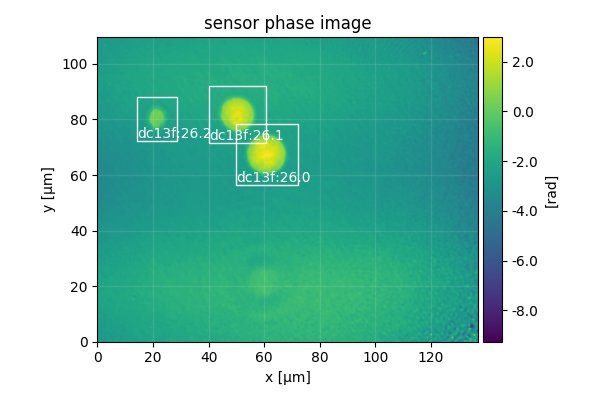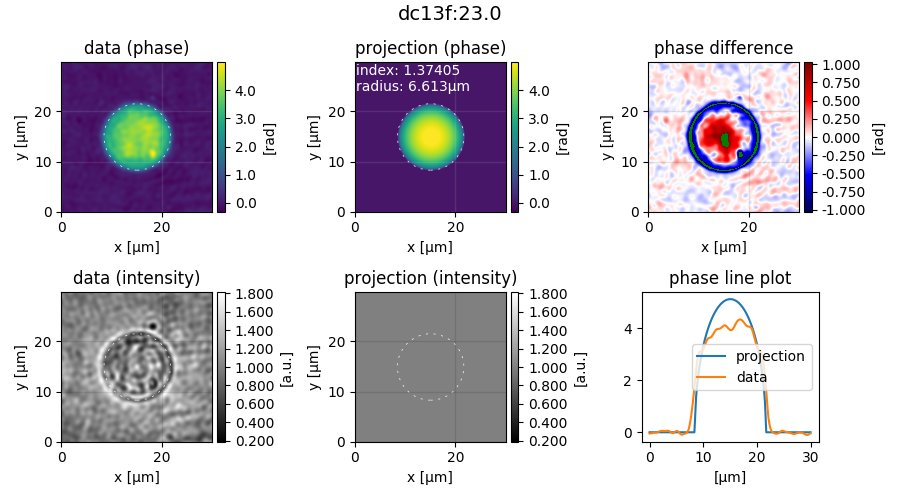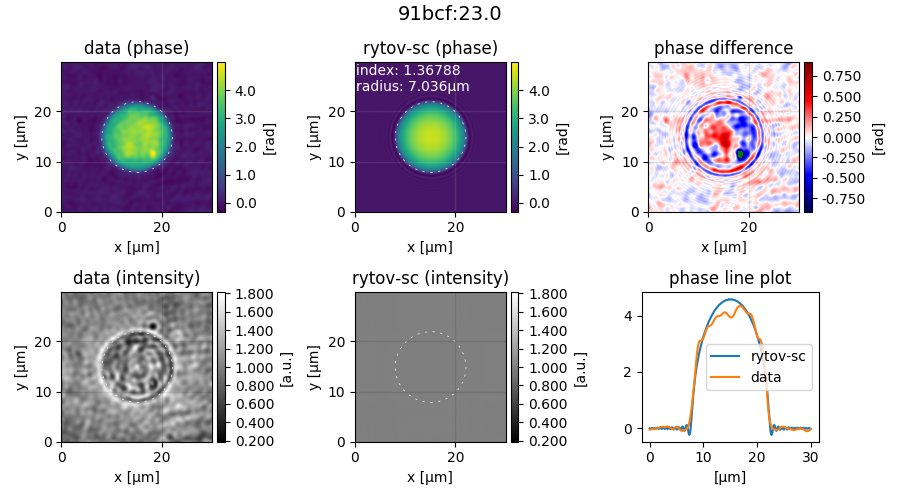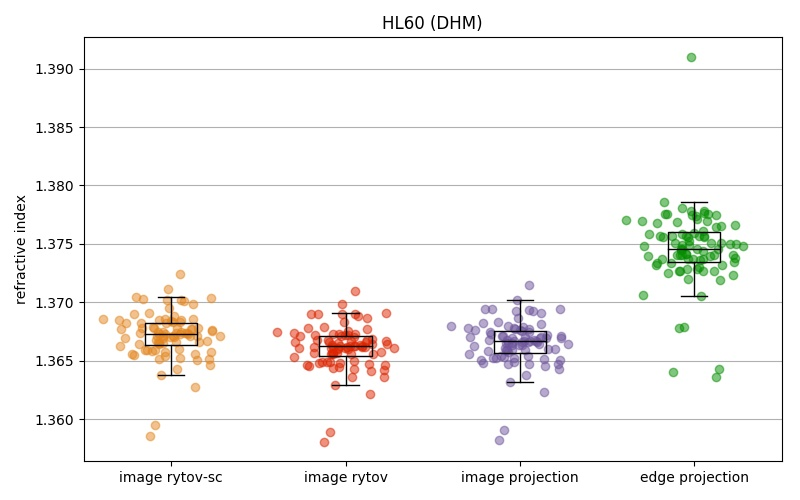T2: HL60 cell analysis (CLI)¶

Introduction¶

HL60 cells in suspension are inhomogeneous, almost-spherical objects. To estimate an average refractive index (RI) of an HL60 cell population, the DryMass command dm_analyze_sphere can be used. This tutorial reproduces data presented in figure 5d of reference [MSG+18].

Prerequisites¶

For this tutorial, you need:

Find regions of interest¶

Note

You can skip this part by copying roi_slices.txt from DHM_HL60_cells.zip into the DHM_HL60_cells.zip_dm folder, running dm_convert and manually adding the [roi] section to drymass.cfg with enabled = False.

We proceed slightly different than in tutorial 1. Before we use the command dm_analyze_sphere to extract the RI values of the HL60 cells, we have to modify our configuration. We start by executing dm_extract_roi DHM_HL60_cells.zip which prompts us for the pixel size (0.107µm), and the wavelength (633nm), which can be found in the readme.txt file inside the zip archive. This command imports the raw data and searches for cells in the phase data. Opening the file sensor_roi_images.tif, we realize that the search parameters are not set optimally. This is image 27:We want to exclude small ROIs and ROIs with a large overlap. Furthermore, we want to include all large cells (see e.g. image 39). Thus, we change the following configuration keys in drymass.cfg:

[specimen]
size um = 13           # approximate cell diameter we are looking for [µm]

[roi]
pad border = 80        # increase border size around cells
size variation = 0.2   # do not allow large variations of specimen size
exclude overlap = 100  # exclude ROIs with an overlap > 100px

With the new configuration, we run dm_extract_roi DHM_HL60_cells.zip again. Now all cells are detected. However, we want to exclude a few due to artifacts or shape issues. To achieve that, we disable the automatic search for ROIs in drymass.cfg

[roi]
enabled = False        # use existing roi_slices.txt

and remove the undesired ROIs from roi_slices.txt, which are 7.3, 14.1, 17.1, 17.2 and 34.1. There should now be a total of 87 ROIs.

Set 2nd order polynomial background correction¶

The default setting for background correction in DryMass is tilt which means that all phase data are corrected by fitting a 2D tilt image to the image borders. For the present dataset, a second order polynomial fit is a better approach, because the background phase does not follow a linear trend. Thus, we choose the poly2o profile and additionally set the fitting border width to 30 pixels. These are the updated lines in the [bg] section of drymass.cfg:

[bg]
phase border px = 30
phase profile = poly2o

Perform sphere analysis¶

We now run dm_analyze_sphere DHM_HL60_cells.zip and are asked to enter the RI of the medium (1.335). By default, the RI of the cells is computed according to [SSM+15]. The following files are created during this step:

• sphere_edge_projection_data.h5: QPI data
• sphere_edge_projection_images.tif: data visualization
• sphere_edge_projection_statistics.txt: results

Note

Warnings about slice and QPImage identifiers can safely be ignored. Setting the RI of the medium changes the internal ROI identifiers. Since we have fixed the ROIs, the identifiers do not match anymore, but the enumeration is still correct.

Let’s have a look at the visualization of ROI 23.0 in sphere_edge_projection_images.tif.The first column shows the experimental data, the second column shows the modeled data (with the cell perimeter indicated by a dashed circle), and the third column contains a residual image (pay attention to the colorbar, green means that the values are outside of the displayed range) and a line plot through the center of the cell. What is most striking about these data is that the RI is overestimated while the radius is underestimated by the edge-projection model. The explanation is that the radius of the cell is determined with an edge-detection algorithm applied to the phase image. Since the edge-detection algorithm determines the edge on the slope of the phase profile and not where the phase profile starts to deviate from the background, it underestimates the radius. The solution to this problem is to take into account the full phase image when determining RI and radius [KKL+07] [MSG+18].

This can be achieved by modifying the [sphere] section of drymass.cfg. In figure 5d of reference [MSG+18], multiple RI-retrieval methods are applied and compared for the same cell population. To repdroduce these data, we run dm_analyze_sphere DHM_HL60_cells.zip three more times with a modified [sphere] section (note that this may take a while).

• Run 1: phase image fit with a projection model

[sphere]
method = image
model = projection

which produces the files

• sphere_image_projection_data.h5
• sphere_image_projection_images.tif
• sphere_image_projection_statistics.txt
• Run 2: phase image fit with the Rytov approximation

[sphere]
method = image
model = rytov

which produces the files

• sphere_image_rytov_data.h5
• sphere_image_rytov_images.tif
• sphere_image_rytov_statistics.txt
• Run 3: phase image fit with the systematically corrected Rytov approximation

[sphere]
method = image
model = rytov-sc

which produces the files

• sphere_image_rytov-sc_data.h5
• sphere_image_rytov-sc_images.tif
• sphere_image_rytov-sc_statistics.txt

Note

We omitted the case model = mie-avg which is part of figure 5d in reference [MSG+18], because of the long fitting time.

To verify that the full-phase-image-based approaches indeed yield lower residuals than the edge-detection approach, let’s have a look at ROI 23.0 of sphere_image_rytov-sc_images.tif.The phase difference and the phase line plots look much better now. Observed deviations mostly originate from the inhomogeneity of the cell.

Plot the results¶

To plot the results, we use the following Python script.

 1 2 3 4 5 6 7 8 9 10 11 12 13 14 15 16 17 18 19 20 21 22 23 24 25 26 27 28 29 30 31 32 33 34 35 36 37 38 39 40 41 42 43 import matplotlib.pylab as plt import numpy as np def dot_boxplot(ax, data, colors, labels, **kwargs): """Combined box and scatter plot""" box_list = [] for ii in range(len(data)): # set same random state for every scatter plot rs = np.random.RandomState(42).get_state() np.random.set_state(rs) y = data[ii] x = np.random.normal(ii+1, 0.15, len(y)) plt.plot(x, y, 'o', alpha=0.5, color=colors[ii]) box_list.append(y) ax.boxplot(box_list, sym="", medianprops={"color": "black", "linestyle": "solid"}, widths=0.3, labels=labels, **kwargs) plt.grid(axis="y") if __name__ == "__main__": ri_data = [ np.loadtxt("sphere_image_rytov-sc_statistics.txt", usecols=(1,)), np.loadtxt("sphere_image_rytov_statistics.txt", usecols=(1,)), np.loadtxt("sphere_image_projection_statistics.txt", usecols=(1,)), np.loadtxt("sphere_edge_projection_statistics.txt", usecols=(1,)), ] colors = ["#E48620", "#DE2400", "#6e559d", "#048E00"] labels = ["image rytov-sc", "image rytov", "image projection", "edge projection"] plt.figure(figsize=(8, 5)) ax = plt.subplot(111, title="HL60 (DHM)") ax.set_ylabel("refractive index") dot_boxplot(ax=ax, data=ri_data, colors=colors, labels=labels) plt.tight_layout() plt.show()Discussion¶

The above figure correctly reproduces the message conveyed with figure 5d of reference [MSG+18]. There are only minor differences that can be explained by a slightly different analysis pipeline:

• In [MSG+18], 84 cells were analyzed as opposed to the 87 cells shown here. This can be attributed to the improved object detection pipeline introduced in DryMass 0.1.4.
• In [MSG+18], the phase data were background-corrected with background data (not included in DHM_HL60_cells.zip) and a linear model (phase profile = tilt) as opposed to a second order polynomial model (which was introduced in DryMass 0.1.3). However, this does not seem to have any significant effect on the results, which indicates that the analysis methods are robust.
• There is a prominent outlier in the edge projection results set. The reason for this outlier is a falsely detected contour (see ROI 1.0). This ROI was not included in the analysis of [MSG+18].
• Other minor differences might originate from the fact that the hologram data is processed differently ([holo] section of drymass.cfg). In [MSG+18], a gaussian filter is used whereas DryMass defaults to a disk filter. For more information on this topic, see e.g. Hologram filter choice.